## 1 Introduction

Wave energy is one of the few untapped sources of renewable energy that could make a significant contribution to the future energy system. Unfortunately, to date, none of the more traditional prototypes which use buoyancy or diffraction wave forces have proven themselves to be commercially viable. This motivates the development of new approaches to wave energy conversion. One of the recent and most promising methods is obtaining energy from the elliptical motion of water wave particles using a horizontal cyclorotor with hydrofoils. Even though this idea is more than 40 years old (McCormick 1979), few prototypes have ever been built and tested (Hermans et al. 1990; Scharmann 2014; Siegel 2019). The authors of these concepts consider different control strategies and models to satisfy their selected performance metrics. In this work, we have derived the new formulae and methods which can significantly simplify the calculation of the previous models and provide a basis for new model-based control design.

### 1.1 Overview of the existing prototypes and control strategies

The first prototype concept of a lift force-based WEC, a rotor with a single hydrofoil Rotating Wing, was tested by Hermans et al. (1990) in the deep water basin of the Maritime Research Institute, in the Netherlands (MARIN). It was shown that the device rotates at the wave frequency and can absorb energy from waves. Subsequent researchers (Scharmann 2014; Siegel 2019) noted that it is difficult to imagine operating this concept in real panchromatic and multi-directional waves without a control strategy.

Significant work on the development of the cyclorotor-based WEC was conducted by the Atargis Energy Corporation (Atargis 2020). The proposed cycloidal wave energy converter (CycWEC) is a cyclorotor with two hydrofoils. This concept was tested as a 1:300 scale prototype, in a 2D wave tunnel of the US Air Force Academy (Siegel et al. 2011a, 2012a, b) and as a 1:10 model in a 3D wave tank at the Texas A&M Offshore Technology Research Center (Fagley et al. 2012a; Siegel et al. 2012a, b). The performance metrics which were proposed for this device are based on the radiated waves or the difference between measured upstream and downstream far-field free surface elevation. Generally, they are based on the ability of the rotating rotor to generate waves with the opposite phase to incoming waves. As a result, it is possible to observe the wave absorption effect downstream, and presume that all the wave’s energy was absorbed by the WEC. Atargis developed a linear feedforward control algorithm, which was used to adjust the shaft angular velocity and rotor position as well as foil pitch angles (Fagley et al. 2012b).

Performance metrics and a control strategy were also proposed in the PhD thesis of Scharmann (2014), where performance metrics are calculated by direct measurement of generator torque and speed. The experiments were conducted in the Hamburg Ship Model Basin, Germany, and have show n that a two-foil rotor will have highly fluctuating torques, since the condition of orthogonality between rotational and wave particle velocities cannot be maintained without allowing discontinuous rotor displacements. This would make efficient conversion from mechanical power to electrical power demanding. The author proposed the concept of the cyclorotor with four hydrofoils and a robust control scheme as the most promising approach.

The reviews of cyclorotor-based WECs conducted by different authors (Scharmann 2014; Folley and Whittaker 2019; Ermakov and Ringwood 2021) have shown that a cyclorotor may have a wide range of possible actuators and may be suitable for different strategies of wave energy extraction. A significant analysis of lift-based wave energy converters and their potential was subsequently conducted by Folley and Whittaker (2019). A classification was developed, based on the specific method of generating lift and the motion of the body. The work reported in Folley and Whittaker (2019) provided the inspiration for the LiftWEC project (2020), which is also dedicated to cyclorotor-based WEC development.

### 1.2 Overview of modelling approach

We present a new analytical model, which can provide the basis for the control design of a cyclorotor-based wave energy converter. Our model is derived for a horizontal cyclorotor with N hydrofoils. It is relatively simple, fast, and suitable for control design. This was achieved using new analytical formulae, which were derived by the authors and validated with the numerical and experimental results, which were published in previous research (Hermans et al. 1990; Siegel et al. 2011a, b). These new formulae significantly decrease the calculation time and increase the accuracy of the results, as well as providing useful insight into the nature of the system behaviour. Our model is suitable for the derivation and testing of new control methods and supports various performance metrics. The developed model is very convenient for control design, using power take off (PTO) torque and hydrofoil pitch angles as control inputs.

Our Sect. 2 presents the mechanical model which is based on Newton’s second law for rotation, and balances the product of the rotor’s acceleration and inertia with the torques caused by the lift and drag forces generated on the hydrofoils. It also introduces the two most direct real-time control inputs: PTO torque and pitch angle.

The hydrodynamic model is described in Sect. 3. The cyclorotor rotation is considered in a two-dimensional potential field of incoming monochromatic waves, and waves generated by the rotating foils. New equations for the free surface elevation, caused by the rotating foils, and corresponding fluid velocities resulting from incoming and radiated waves, are presented.

In our Sect. 4, we present the validation of the new formulae with the results of previous research for far-field free surface elevation. Robust agreement with numerical and experimental tests was achieved.

Approximate methods for the determination of the lift and drag forces are considered in our Sect. 5. To determine the lift and drag forces, it is necessary to define the interaction between the hydrofoils and the overall relative fluid velocity, consisting of the incoming wave-induced hydrodynamic velocities, velocities of the waves generated by the rotating hydrofoils, and the rotational velocity of the rotor. Two possible models of hydrofoils, as a both point source and a thin chord, are presented.

Our Sect. 6 outlines the numerical methods and solutions for the developed models and some results. The presented Fig. 6 of the angular velocity for free motion illustrate the ability of the rotor to rotate with the frequency of the incoming monochromatic waves.

Our conclusion sums up the presented elements of the model and calculated examples of its application, and discusses future possible applications of the model for various types of rotor and rotor design optimisation.

## 2 Mechanical model of the rotor

We consider wave propagation in the Cartesian coordinate system, and the rotor rotation in polar coordinates Fig. 1. The rotational centre of the cyclorotor is located on the x axis and submerged by $$y_0$$. Then, the position of hydrofoils $$i=1,2,\ldots n$$ can be determined as:

\begin{aligned} x_i(t)= & {} R \cos (\theta (t)+2\pi (i-1)/n) \end{aligned}
(1)
\begin{aligned} y_i(t)= & {} y_0-R \sin (\theta (t)+2\pi (i-1)/n) \end{aligned}
(2)

where $$(x_i, y_i)$$ is the position of hydrofoil i, R is the operational radius, and $$\theta (t)$$ is the polar angle, which determines the position of the foils in the selected time moment $$t_i$$.

Taking the time derivatives of (1) and (2), we can obtain the vector of the rotational velocity $${\mathbf {V}}_{\mathbf {R}}=\{(V_{R})_x,(V_{R})_y\}$$:

\begin{aligned} (V_{R_i})_x= & {} -R\, {\dot{\theta }}(t)\sin (\theta (t)+2\pi (i-1)/n) \end{aligned}
(3)
\begin{aligned} (V_{R_i})_y= & {} -R\, {\dot{\theta }}(t)\cos (\theta (t)+2\pi (i-1)/n) \end{aligned}
(4)

where $${\dot{\theta }}(t)$$ is the angular velocity.

The mechanical model of the rotor is based on Newton’s second law for rotation and balances the product of the rotor’s acceleration and inertia with the torques caused by the tangential forces generated on the hydrofoils. It makes it directly connected with a rotational generator, which exerts an opposing torque, $${\mathcal {T}}$$:

\begin{aligned} I \ddot{\theta }(t)=\sum _{i=1}^{N} F_{T_i} R - {\mathcal {T}} \end{aligned}
(5)

where I—the inertia of the cyclorotor, $$\ddot{\theta }(t)$$—the angular acceleration, $$F_{T_i}$$—tangential forces generated on the hydrofoil i due to the interaction with incoming waves, they can be manipulated by pitching the hydrofoils and changing the attack angle $$\alpha$$. The PTO torque $${\mathcal {T}}$$ is used both to take rotational energy from the system to generate electrical energy, or supply energy to increase rotational speed. In the second case, we presume the ability of PTO to switch to a motoring mode. The balance equation (5) is presented in a basic form. A more advanced model can be obtained by including additional terms which represent the mechanical damping from the shaft which connects the foil and the central PTO, or entrained fluid inertia caused by added fluid mass. Such effects are omitted in this initial treatment, for simplicity.

As an example performance function, we use captured energy in a traditional form used in wind, tide and wave energy metrics. It is defined as maximisation of the time integral of the product between angular velocity $${\dot{\theta }}(t)$$ and PTO torque on the time interval [0, T].

\begin{aligned} J=\int _0^T{\mathcal {T}}(t){\dot{\theta }}(t){\mathrm{d}}t \rightarrow \text {Max}. \end{aligned}
(6)

## 3 The hydrodynamic model

We consider the rotation in two-dimensional potential flow which includes incoming monochromatic or panchromatic waves, as well as radiated waves generated by the rotating rotor, and viscous losses.

As an example of incoming waves, we present Airy waves which were used in the study of Siegel et al. (2011b), which can be described by the following velocity potential:

\begin{aligned} \varPhi _{{\mathrm{W}}}(x,y,t)=\frac{H g}{2\omega }{\mathrm{e}}^{k y}\sin (k x - \omega t) \end{aligned}
(7)

where H is the wave height, $$\omega$$ is the wave frequency, k is the wave number, and g is the acceleration due to gravity.

The components of the wave-induced velocity $${\mathbf {V}}_{{\mathbf {W}}}$$ can be found as a gradient from the potential:

\begin{aligned} {\mathbf {V}}_{\mathbf {W}}=\nabla \varPhi _{{\mathrm{W}}}(x,y,t)=\left\{ \left( V_{{\mathrm{W}}}\right) _x, \left( V_{{\mathrm{W}}}\right) _y\right\} . \end{aligned}
(8)

From these partial derivatives, we get the components of the wave induced velocity:

\begin{aligned} \left( V_{{\mathrm{W}}}\right) _x= & {} \frac{{\mathrm{e}}^{k y} g H k }{2 \omega }\cos (k x-t \omega ) \end{aligned}
(9)
\begin{aligned} \left( V_{{\mathrm{W}}}\right) _y= & {} \frac{{\mathrm{e}}^{k y} g H k }{2 \omega }\sin (k x-t \omega ). \end{aligned}
(10)

One of the challenges in the development of the cyclorotor-based WEC is the estimation of the waves radiated by rotating hydrofoils. In previous works (Hermans et al. 1990; Scharmann 2014; Siegel 2019), the hydrofoil, from the far-field, was modelled as a single moving point vortex in infinitely deep water. This vortex can be represented by a complex potential which satisfies the kinematic and dynamic boundary condition on the free surface (Wehausen and Laitone 1960):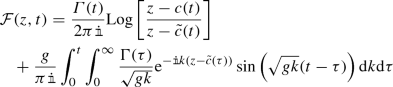(11)

where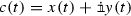is the position of the hydrofoil, $${\tilde{c}}(t)$$ is the complex conjugate of c(t), k is the wave number and $$\Gamma (t)$$—is the circulation of the vortex, or the line integral of the fluid velocity along a closed path.

The potential $${\mathcal {F}}(z,t)$$ in (11) consists of two parts. The first term on the right-hand side of (11) the instantaneous (momentary) radiation and has a singularity at the source point c(t). For this reason, it can not be used to describe the state in the close vicinity of the foil. The second term on the right-hand side of (11) describes the fluid velocity wake caused by the moving vortex. In the study by Hermans et al. (1990), this term is calculated numerically, using double integration over the wave number k and the time parameter $$\tau$$. A very similar approach is employed in the work of Siegel et al. (2011b), where it was integrated using second order k and $$\tau$$ marching techniques.

The authors of this work have solved the integral over k analytically in the form of the Dawson function D[x] (Dawson 1897):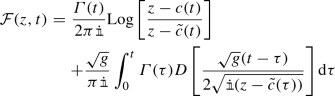(12)

where

\begin{aligned} D(x) = {\mathrm{e}}^{-x^2} \int _0^x {\mathrm{e}}^{y^2} {\mathrm{d}}y. \end{aligned}
(13)

This representation is valid only when $$\text {Im}[z - {\tilde{c}}(\tau )] < 0$$ or $$y + y_i < 0$$, which is always true for the area under consideration, since $$y < 0$$ and $$y_i < 0$$. This new formula significantly decreases the calculation time and increases the accuracy of the results, since all the wave numbers k are now covered and we only need to find one integral with defined limits.

The velocity of the waves radiated by a rotating hydrofoil can be found using the following equation: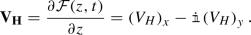(14)

The velocity field $${\mathbf {V}}_{\mathbf {H}}$$ of the waves radiated by the hydrofoil also consists of the instantaneous (momentarily) radiated waves $${\mathbf {V}_{{\mathbf {HM}}}}$$ and wakes $${\mathbf {V}}_{{\mathbf {HW}}}$$ which were left on the hydrofoil’s path:

\begin{aligned} {\mathbf {V}}_{{\mathbf {H}}} = {\mathbf {V}_{{\mathbf {HM}}}} + {\mathbf {V}}_{{\mathbf {HW}}}. \end{aligned}
(15)

The components of the velocity field caused by the hydrofoil i at the point j are:

\begin{aligned} \left( V_{{\mathrm{HM}}}\right) _x&= \frac{\Gamma _i(t) y_i\left( \left( x_j-x_i\right) ^2-\left( {y_j}^2-y_i^2\right) \right) }{\pi \left( \left( \left( x_j-x_i\right) ^2-\left( {y_j}^2-y_i^2\right) \right) ^2 +4\left( {y_j}\left( {x_j}-x_i\right) \right) ^2\right) } \end{aligned}
(16)
\begin{aligned} \left( V_{{\mathrm{HM}}}\right) _y&= \frac{2 \Gamma _i(t) y_j y_i\left( x_j-x_i\right) }{\pi \left( \left( \left( x_j-x_i\right) ^2-\left( {y_j}^2-y_i^2\right) \right) ^2 +4\left( y_j\left( x_j-x_i\right) \right) ^2\right) } \end{aligned}
(17)
\begin{aligned} \left( V_{{\mathrm{HW}}}\right) _x&= - \int _0^t\frac{\Gamma _i(\tau )\sqrt{g}}{2\pi }\cos \left( x_j-x_i[\tau ]\right) \left( -\frac{\sqrt{g}(t-\tau )}{\left( y_j+y_i[\tau ]\right) ^2}\right. \nonumber \\&+\left. \frac{\left( g (t-\tau )^2+2 \left( y_j+y_i[\tau ]\right) \right) }{\left( -y_j-y_i[\tau ]\right) ^{5/2}} D\left[ \frac{\sqrt{g} (t-\tau )}{2 \sqrt{-y_j-y_i[\tau ]}}\right] \right) {\mathrm{d}}\tau \end{aligned}
(18)
\begin{aligned} \left( V_{{\mathrm{HW}}}\right) _y =&\int _0^t\frac{\Gamma _i[\tau ]\sqrt{g}}{2\pi }\sin \left( x_j-x_i[\tau ]\right) \left( -\frac{\sqrt{g} (t-\tau )}{ \left( y_j+y_i[\tau ]\right) ^2}\right. \nonumber \\&+\left. \frac{\left( g (t-\tau )^2+2 \left( y_j+y_i[\tau ]\right) \right) }{\left( -y_j-y_i[\tau ]\right) ^{5/2}}D\left[ \frac{\sqrt{g} (t-\tau )}{2 \sqrt{-y_j-y_i[\tau ]}}\right] \right) {\mathrm{d}}\tau . \end{aligned}
(19)

The complex potential $${\mathcal {F}}(z,t)$$ can also be presented in the form of the sum of the velocity potential $$\varPhi _H$$ and stream function $$\varPsi _H$$ as: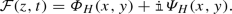(20)

Thus, the new velocity potential for waves radiated by the hydrofoil, which was derived by the authors from (11), using representation (20) and Dawson function (13), has the following form: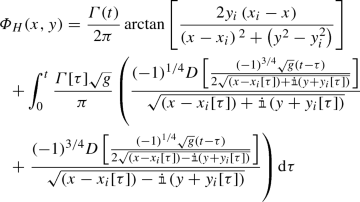(21)

and, despite the presence of the complex terms, the value of the function in (21) is always real. In addition, for cases where multiple (square) roots of a variable are taken, the following development ultimately utilises only the square of the root, making it immaterial which of the roots is taken.

In the case of the potential flow, the free surface perturbation can be found from the dynamic boundary condition. For example, the elevation of the free surface caused by the Airy wave has the following form:

\begin{aligned} \eta _w = -\frac{1}{g}\left( \frac{\partial \varPhi _{{\mathrm{W}}}}{\partial t} \right) _{y=0} = \frac{H}{2}\cos (k x - \omega t). \end{aligned}
(22)

Now, we can obtain the perturbation of the free surface caused by the rotating hydrofoil i using Eq. (21):

\begin{aligned} \eta _{h_i} = -\frac{1}{g}\left( \frac{\partial \varPhi _{H_i}}{\partial t} \right) _{y=0} \end{aligned}
(23)

and the overall elevation of the free surface can be presented in the form of the linear sum:

\begin{aligned} \eta = \eta _w+\sum _{i=1}^{n}\eta _{h_i}. \end{aligned}
(24)

## 4 Model validation via free surface displacement

In this section, we validate the results obtained from (21) and (23) for the heights and periods of the waves generated by a single rotating hydrofoil, against results obtained experimentally and numerically by previous researchers (21).

In the research work by Hermans et al. (1990), the authors derive an analytical equation that can be used to compute the heights of waves generated by a foil which rotates at a constant rate. This equation is only valid for relatively large values of t, i.e. when stable periodic wave generation is achieved. The authors solved the system (11) and (23) numerically and the calculated results were compared with the experimental data. The experiments were conducted in the deep water basin of MARIN. The prototype consisted of a single hydrofoil with chord length $$l = 0.1$$ m, operating radius $$R = 0.14$$ m, submerged depth of $$y_0 = -0.271$$ m with the wave profile measured at a point located at $$x = 1.8$$ m. The circulation was defined as $$\Gamma =\pi |\omega R| \,l \, \tan (\alpha )$$. Figure 2b shows the published results from Hermans et al. (1990) for the free surface elevation at the measurement point for a foil rotating with $$\omega = 6.91$$ rad/s and $$\alpha = 0.576$$ rad. It can be seen that good agreement between the amplitude and period of the radiated waves was obtained Fig. 2.

In total, two validations were conducted with the results published in the works of Siegel et al. (2011a, 2011b). The first case corresponds to the results of the 1:300 scale experiment which was conducted in the 2D wave tunnel of the US Air Force Academy (Siegel et al. 2011a). Figure 3b presents the upstream (blue line) and downstream (green line) free surface elevation caused by the single rotating hydrofoil. The experimental setup has the following parameters: rotor rotation period $$T_{\mathrm{r}} = 0.55$$ s, blade pitch $$\alpha = -7.5^{\circ }$$, operational radius $$R = 6~\text {cm},$$ chord length $$S = 5$$ cm, submergence $$y_0 = -7.5$$ cm. We have determined the lift coefficient as $$C_{\mathrm{L}} = -0.65$$ and the circulation as $$\Gamma = C_{\mathrm{L}} \omega R S /2$$. The numerical simulation, with the use of new formulae, shows good agreement with the amplitude and period of the experimentally radiated waves, as seen in Fig. 3.

The last validation case is the comparison with the numerical simulation presented in Siegel et al. (2011b). The parameters were normalised by a period of $$T = 9$$ s and wave length $$\lambda _{{\mathrm{Airy}}} = 126.5$$ m. The single hydrofoil rotor has radius $$R/\lambda _{{\mathrm{Airy}}} = 0.15,$$ submergence depth $$|y_0 |/\lambda _{{\mathrm{Airy}}} = 0.18$$, and circulation $$\Gamma T/{\lambda ^2_{{\mathrm{Airy}}}} = 5.6*10^{-3}$$. All waves are evaluated at $$x = \pm 3 \lambda _{{\mathrm{Airy}}}$$ and at time $$t/T = 30$$ after the start of the cycloidal WEC.

The numerical results obtained in Siegel et al. (2011b) are presented in Fig. 4b, where the upstream (black line) and downstream (grey line) are in very good agreement with our numerical results, shown in Fig. 4a.

Figures 3 and 4 also show that the amplitude of upstream radiated waves (blue and grey lines) are more than ten times less than the amplitude of the waves radiated downstream (green and black lines). Assuming that the downstream radiated waves have the same amplitude as incoming wave, but opposite phase, we can achieve complete wave energy absorption (Siegel 2019). As a result, the interaction between the upstream radiated waves and the incoming waves can be ignored, due to the significant amplitude differences. This effect allows us to reliably forecast the wave-induced fluid velocity. Thus, the derived equations (21) can be very beneficial for the calculation of the most recent performance metrics proposed by Siegel (2019), which are based on wave radiation and cancellation effects.

## 5 Approximate determination of lift and drag forces

Modelling of the hydrofoil interaction with the wave velocity field is a challenging problem. Accurate determination of the attack angle, circulation, lift and drag forces requires significant high-fidelity computation, such as computational fluid dynamics (CFD). All these do not make the high-fidelity models suitable for control design. In this section, we consider two possible approximate models for lift and drag forces which can be used for the control design.

### 5.1 Point source representation

This is a very basic representation which considers the hydrofoils as point sources. For this case, the lift and drag coefficients should be considered not as physical values, but more as tuning parameters. These best-fit approximate coefficients can be obtained from numerical simulation or experimental tests.

These parameters depend on the system inputs, which can be measured or tracked in real time:

1. 1.

The rotational velocity $${\mathbf {V}}_{{\mathbf {R}}}$$ and position of the rotor $$\theta$$ can be measured and controlled

2. 2.

The wave-induced fluid velocity $${\mathbf {V}}_{{\mathbf {W}}}$$ can be reliably predicted in real time due to the minimal upstream radiation

3. 3.

The velocity of waves radiated by the hydrofoils $${\mathbf {V}}_{\mathbf {H}}$$ can be calculated relatively easily; however, we cannot define the instantaneous radiated waves $$({\mathbf {V}_{{\mathbf {HM}}})}_i$$ in the small vicinity of the point source i, due to the singularity highlighted in Sect. 3.

Thus, we consider the generation of the lift and drag forces as the result of the rotation of the hydrofoil i with an overall relative velocity $${\hat{\mathbf {V}}}_{\mathbf {i}}$$, representing the vector difference between the wave-induced fluid velocity $${\mathbf {V}}_{{\mathbf {W}}_{\mathbf {i}}}$$ and the cyclorotor rotational velocity $${\mathbf {V}}_{{\mathbf {R}}_{\mathbf {i}}}$$, plus the sum of the wakes caused by the hydrofoil rotation $${\mathbf {V}}_{{\mathbf {HW}}}$$ and instantaneous radiation from the other foils $${\mathbf {V}_{{\mathbf {HM}}}}$$ as:

\begin{aligned} {\hat{\mathbf {V}}}_{\mathbf {i}} = {\mathbf {V}}_{{\mathbf {W}}_{\mathbf {i}}} - {\mathbf {V}}_{{\mathbf {R}}_{\mathbf {i}}} + \sum _{j}^{N \setminus i}{\mathbf {V}}_{{{\mathbf {HM}}}_j} + \sum _{j}^{N}{\mathbf {V}}_{{{\mathbf {HW}}}_j}. \end{aligned}
(25)

The attack angle $$\alpha _i(t)$$ can be found as the angle between the cyclorotor rotational velocity $${\mathbf {V}}_{{\mathbf {R}}_{\mathbf {i}}}$$ and overall relative velocity $${\hat{\mathbf {V}}}_{\mathbf {i}}$$:

\begin{aligned} \alpha _i(t) = \arcsin \left( \frac{(V_{R_i})_x * ({\hat{V}}_i)_y - (V_{R_i})_y * ({\hat{V}}_i)_x}{|V_{R_i}| |{\hat{V}}_i|}\right) + \gamma _i \end{aligned}
(26)

where $$\gamma _i$$ is the hydrofoil pitch angle, which can be adjusted in real time.

For the point source representation, we use the following approximation: $$F_{\mathrm{L}}$$ lift and $$F_{\mathrm{D}}$$ drag forces which act on a particular hydrofoil depend on the lift and drag coefficients $$C_{\mathrm{L}}(\alpha )$$ and $$C_{\mathrm{D}}(\alpha )$$, hydrofoil chord length S, fluid density $$\rho$$ and overall relative velocity $${\hat{\mathbf {V}}}$$ at a hydrofoil position $$\left( x_i,y_i\right)$$:

\begin{aligned} F_{\mathrm{L}}= & {} \frac{1}{2} \, \rho \, C_{\mathrm{L}} |{\hat{V}}|^2 \, S, \end{aligned}
(27)
\begin{aligned} F_{\mathrm{D}}= & {} \frac{1}{2} \, \rho \, C_{\mathrm{D}} |{\hat{V}}|^2 \, S. \end{aligned}
(28)

The circulation $$\Gamma$$ can be determined using the following equation:

\begin{aligned} \Gamma = \frac{1}{2} \,C_{\mathrm{L}} \, |{\hat{V}}| \, S. \end{aligned}
(29)

The tangential force $$F_{\mathrm{T}}$$ can now be presented as a combination of the lift $$F_{\mathrm{L}}$$ and drag $$F_{\mathrm{D}}$$ forces:

\begin{aligned} F_{\mathrm{T}} = F_{\mathrm{L}}(\alpha ) \sin \left( \alpha -\gamma \right) - F_{\mathrm{D}}(\alpha ) \cos \left( \alpha -\gamma \right) . \end{aligned}
(30)

### 5.2 Thin-chord hydrofoil representation

A more advanced approach than the point source model describes the hydrofoil as a thin chord. We use the vortex panel representation described in Katz and Plotkin (2001) for an unsteady thin airfoil using the lumped-vortex element method. This method was employed in Siegel et al. (2011b) for a thin hydrofoil panel representation in order to analyze the near field of the hydrofoil. The foil chord is divided into m linear segments $$\varDelta l_i$$, with the boundaries of these segments shown by the black points in Fig. 5. The lump-vortex is placed at each quarter segment (i.e. $$1 /4\varDelta l_i$$) with collocation points placed at three quarters of each segment (i.e. $$3/4 \varDelta l_i$$). We have selected the lumped-vortex element in the form of the instantaneous radiation $${\mathbf {V}_{{\mathbf {HM}}}}$$ Eqs. (16) and (17) which satisfies the free surface condition, where $$\{x_i,y_i\}$$ are the coordinates of the vortex position and $$\{x_j,y_j\}$$ are the coordinates of the collocation points. This vortex panel representation allows us to define the fluid circulation $$\Gamma _i$$ in the vicinity of the foil and, as a result, the pressure difference $$\varDelta p_i$$ between the panel sides. The additional $$m+1$$ point is added, at the trailing edge of the foil, to calculate the strength of the wake vortex left by the hydrofoil at each time step. For each segment, the normal $${\mathbf {n}}_i$$ and tangential $${\varvec{\tau }}_i$$ vectors are defined.

The following system of linear algebraic equations is used to define the circulation components $$\Gamma _i$$:

\begin{aligned} \sum _{i=1}^{m+1} \Gamma _i \, \left( {\mathbf {V}_{{\mathbf {HM}}}}_{(i,j)} \cdot {\mathbf {n}}_i \right) = - ({\hat{\mathbf {V}}}_i \cdot {\mathbf {n}}_i) \quad j=1\ldots m \end{aligned}
(31)

with the additional $$m+1$$ equation representing the Kelvin condition:

\begin{aligned} \sum _{i=1}^{m+1} \Gamma _i = \Gamma (t-\varDelta t) \end{aligned}
(32)

where $$\Gamma (t-\varDelta t)$$ is the circulation measured at the previous time step.

The solution of the system (31) and (32) determines the values of the circulation $$\Gamma _i$$ on each of the intervals $$\varDelta l_i$$, the overall circulation caused by the hydrofoil:

\begin{aligned} \Gamma = \sum _{i=1}^m \Gamma _i \end{aligned}
(33)

and intensity of the wake $$\Gamma _{m+1}$$.

The difference between pressures on proximal and distal surfaces (relative to the axis of rotation) of the segment $$\varDelta l_i$$ can be obtained from:

\begin{aligned} \varDelta p_i = \rho \left[ \frac{\Gamma _i}{\varDelta l_i} \left( {\hat{\mathbf {V}}}_i\cdot {\varvec{\tau }}_i \right) + \frac{\partial }{\partial t} \sum _{j=1}^i\Gamma _j \right] . \end{aligned}
(34)

Thus, the lift and drag forces can be defined from (34) as:

\begin{aligned} F_{\mathrm{L}} = \sum _{i=1}^m \varDelta p_i \varDelta l_i \cos {\beta _i}, \end{aligned}
(35)
\begin{aligned} F_{\mathrm{D}} = \sum _{i=1}^m \varDelta p_i \varDelta l_i \sin {\beta _i} \end{aligned}
(36)

where $$\beta _i$$ is the angle between the segment and the tangent to the rotation trajectory, drawn through the connection point of hydrofoil chord and operational radius R. The total tangential force can now be defined using Eq. (30).

The system (27), (28), (35) and (36) can be solved for $$C_{\mathrm{L}}$$ and $$C_{\mathrm{D}}$$ and the corresponding angle of attack can be found from Eq. (26). However, due to the complex, non-homogeneous fluid velocity field in the vicinity of the hydrofoil, the system does not have a unique solution for lift and drag coefficients at the single point source where the attack angle is defined. The determination of $$C_{\mathrm{L}}$$ and $$C_{\mathrm{D}}$$, and the corresponding angle of attack, for the selected point on the foil requires a number of simulations runs to find the best statistical fit for these coefficients e.g. using least squares.

## 6 Numerical solution methods and some results

In this section, we present the results of numerical simulations obtained with the use of the point source and thin-chord representations already outlined in Sects. 5.1 and 5.2, respectively. Our main goal is to illustrate the capabilities of the developed models and assess their possible applicability to control design. As an example, we consider the rotor with two hydrofoils which is similar to the CycWEC prototype tested by Atargis in a 3D wave tank at the Texas A&M Offshore Technology Research Center. The selected rotor has foils with chord length $$S = 0.75$$ m, operational radius $$R = 1$$ m, submergence depth $$y_0 = 2$$ m, inertia $$I = 500~\text {kg}~\text {m}^2$$.

We present an asymmetric rotor, with pitch angles $$\gamma _1 = 7^{\circ }$$ and $$\gamma _2 = -7^{\circ }$$. This configuration ensures convergence with the wave frequency and achievement of a stable periodic solution. Unfortunately, the results presented in Siegel (2013) do not allow us to reliably determine the lift (and especially) drag coefficients for the curved hydrofoils of type NACA0015, which were tested in these very specific conditions. We have selected the lift and drag coefficients using the point source method from the publicly available (Sheldahl and Klimas 1981) for symmetric thinner hydrofoils of type NACA0012 for $$\text {Re} = 2 \times 10^6$$. The similar value was used in Ansys simulations of the CycWEC conducted by Caskey (2014). The thinner NACA0012 hydrofoils allow us to have a better agreement with the thin-chord model. For the case of the thin-chord profile, the camber which can be described by equation for the shape of a four-digit NACA foil NASA (2020) was taken:

\begin{aligned} y = 0.2969 \sqrt{x}-0.1260 x - 0.3516 x^2 + 0.2843 x^3 - 0.1015 x^4 \end{aligned}
(37)

where $$x \epsilon [0,1].$$

We consider the free motion in monochromatic waves with height $$H = 0.9$$ m, length $$L = 9.75$$ m and period $$T = 2.5$$ s. Initially, the hydrofoil rotor’s chord is parallel to the free surface, i.e. $$\theta (0) = 0$$, and the angular velocity is equal to the wave frequency, i.e. $${\dot{\theta }}(0) = \omega$$.

A discrete-time is considered and the time period T is separated into the set of n small intervals $$\varDelta t_i = \left\{ t_i,t_{i+1}\right\}$$. It is assumed, that at each small interval $$\varDelta t_i$$, the rotational velocity $${\dot{\theta }}_i$$ is constant. Then, the kinematic parameters of the rotor, at the next time step, can be found from this scheme:

\begin{aligned} \theta _{i+1}= & {} \theta _i+{\dot{\theta }}_i \varDelta t_i + {\ddot{\theta }}_i {\varDelta t_i}^2/2 \end{aligned}
(38)
\begin{aligned} {\dot{\theta }}_{i+1}= & {} {\dot{\theta }}_i+{\ddot{\theta }}_i \varDelta t_i, \end{aligned}
(39)
\begin{aligned} {\ddot{\theta }}_{i+1}= & {} \left( \sum _{i=1}^n{F_{\mathrm{T}}}_i[\Gamma _i,{\theta }_{i},{\dot{\theta }}_{i},\alpha _i]\,R - {\mathcal {T}}_i\right) /I. \end{aligned}
(40)

All the results were obtained using the finite difference method for differentiation and the trapezoidal rule for integration. All calculations were conducted in Wolfram Mathematica. It takes 4 and 103 s, in real time, to simulate a 1-min scenario for the point source model and the thin-chord model, respectively, using a laptop with 6 cores, running at 2.2 GHz.

The results of the calculations are presented in Figs. 6 and 7. We can see that the angular velocity $${\dot{\theta }}$$ starts to converge with the wave frequency $$\omega$$. Stable periodic rotation was achieved, and the point source and thin-chord model transient behaviours are synchronised with the wave period T after 150 s.

The curved hydrofoils, represented by the thin-chord model (red line), experience much less fluctuation of the torque sign (Fig. 7) than the straight foils (blue line) represented by the point source model. It can be seen that the changes of the torque value are in agreement with the incoming wave periods. This effect can be observed as the additional low frequency content for the curved foils on Fig. 7a (red line). We can also see more significant torque value fluctuation for the point source model in Fig. 7a (blue line). These changes cause the notable low frequency content for the point source model rotational rate shown in Fig. 6a. It can be concluded that straight foils are much more sensitive to fluid velocity field changes caused by incoming waves.

The visible difference in the angular velocity amplitudes (Fig. 6b) can be resolved by adjusting the values of lift and drag coefficients, which were originally obtained for aerofoils in unidirectional flow. This is a simple task due to the linear dependence of the torque on $$C_{\mathrm{L}}$$, $$C_{\mathrm{D}}$$. The authors obtained acceptable agreement of the amplitudes predicted by the point source and chord models by multiplying the coefficients, determined for the aerodynamic case, by 0.6 (as a tuning parameter). However, these fitting coefficients will work only for this particular scenario, and a more advanced study of the best fit coefficients, across a broader range of operational conditions, is needed. Despite the hypothetical nature of the presented lift and drag coefficients, we can presume that the real value of these foil coefficients, with rotation in omnidirectional fluid flow, should be significantly smaller than the values which were obtained in the ideal conditions of aero tubes for unidirectional flow.

## 7 Conclusion

The developed models are validated and fast lending themselves to the control design. For example, the point source model is suitable for model predictive control (Faedo et al. 2017), since it takes approximately 4 s to calculate a 1-min forecast. Customised coding would likely reduce this computational time by an order of magnitude. However, the conducted numerical simulations have shown that the lift and drag coefficients for hydrofoils should be smaller than the coefficients obtained for aerofoils. Thus, lift and drag coefficients should be defined for the selected operational conditions using the thin-chord model, or from more accurate CFD simulations. The model is suitable for development of various control strategies which target different performance metrics, such as the wave cancellation proposed by Siegel (2019) or the maximisation of the power coefficient (6) proposed by Scharmann (2014). The new derived exact analytical formulae for free surface elevation, and perturbation in fluid velocity, caused by a rotating foil can also help developers of existing and new cyclorotor concepts. The presented equations can describe rotors with various numbers of foils and configurations, leading to a potential use in the optimisation of cyclorotor design.